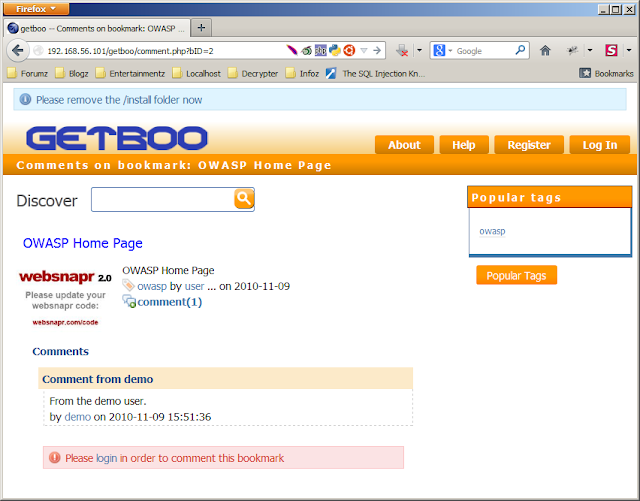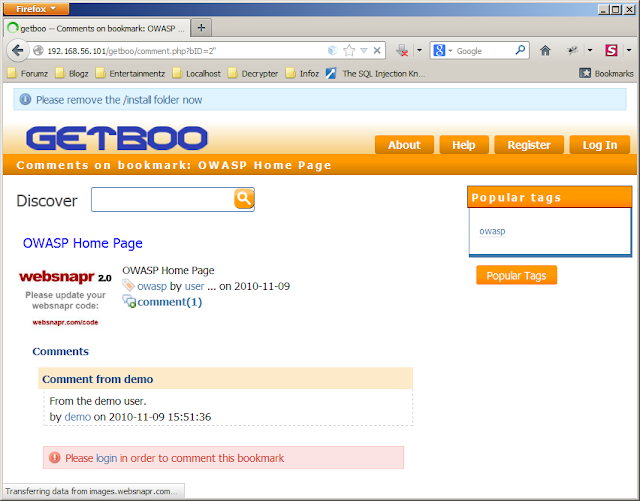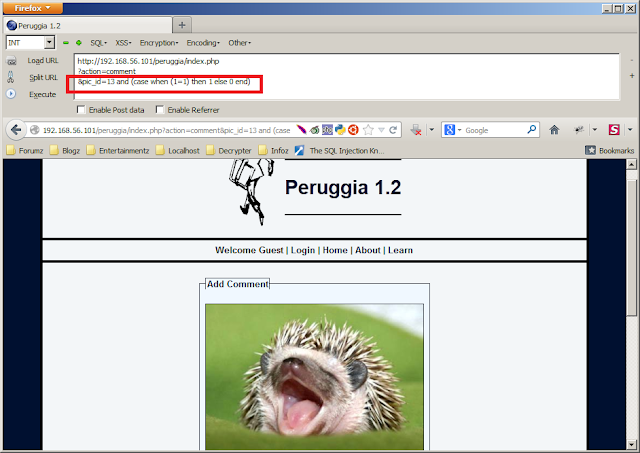## Blind SQLi Detection and How to Inject it

Hello all,

So, today I want to share with you guys on how to detect if the website is vulnerable to Blind SQLi or not.
Before, do note that I'm not an expert in this security/hacking scene. This sharing based on my own understanding from articles/discussions among of these great people

• Mario Heiderich
• Miroslav Stampar
• R4x0r4x
• Nurfed
• Reiners
• benzi
• and more :)
In this article, I'll use OWASP Broken Web Applications Project as example. You guys can get it Here. Hope you guys already have a basic in SQLi so this wont be hard on you guys :
Ok lets move on.
In Blind SQLi, we need to understand correctly on how the server/website response based on TRUE or FALSE condition.There are 2 ways (afaik based on my knowledge) to detect it.

1. Quotes
It can be either single quote (') , double quotes (")  or backtick ( ` )
Refer example below.A normal page condition ( TRUE condition )The page become blank (FALSE condition) once we put a single quoteThe page back to normal condition (TRUE) once we put another single quote.
We can use these method as well to check the TRUE/FALSE condition under this way of detection.

2. Numeric Operators
Use a simple math! Check the example shown below where pic_id is vulnerable to SQLiNormal page loaded. Because the condition is true. 1=1 is TRUEThe admin word is missing. This shows a FALSE condition since 1=2 is FALSE.Another way is by using simple calculation. The current page loaded fine on pic_id=13.The page loaded fine but it shows another page. This is because we added 1 in the pic_id where it'll become 13+1=14 so the page will loaded the pic_id=14 Here are some other method under this technique.

So, how are we going to proceed with our Blind SQLi?
Each dbms have a different techniques but quite similar. In this article, I'll show some common technique used in MySQL dbms.

i. Common technique
id=1 and 1=1
id=1 and (put our sql query here)=(put our expectation here)
as  example we want to query the current version,
id=1 and substring(@@version,1,1)=4
so, if the current MySQL version used by the website started with 4 the page will load fine (TRUE condition) else the page will be error/blank (FALSE condition)

Example shown below.Testing if the MySQL used is version 4.*. Page error,shows that the website is not using that version.Testing if the MySQL used is version 5.*. Page loaded fine,shows the current version used is 5.*

ii. Using a Case statement
id=1 and 1
id=1 and (CASE when (our sql query here) THEN 1 else 0 END)
If the query is TRUE,it'll resulting 1 where 1 is TRUE condition. Else, it'll resulting 0 where 0 is FALSE condition.1=2 is wrong,so it'll resulting 0,FALSE.1=1 is correct,so it'll resulting 1,TRUE.
iii. Time Based
Yup,this is another technique of Blind SQLi based on the server time response.
This technique already explained in my previous post here

and there are more techniques in SQLi out there. Do have some read and research on them as well. You might find a new way on exploiting, who knows right? :D